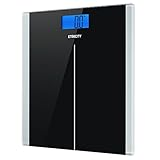ContentsWeight

### Tags

Weight

Below is the degree of change seen after Weight is higher than average.

Below is the change in Weight seen after the predictor is higher than average.

Outcomes
of
Below is the change in the listed outcome after is higher than average.
Sort by Effect Size
Sort by Confidence
% Change from Baseline*
*
Change in the listed outcome after is higher than average.
Predictors
of
Below is the change in after the listed predictor is higher than average.
Sort by Effect Size
Sort by Confidence
% Change from Baseline*
*
Change from the average seen after the predictor is higher than average.

### Weight Info

Property Value
Variable Name Weight
Aggregation Method MEAN
Analysis Performed At 2020-10-09
Duration of Action 7 days
Kurtosis 2.6505359056344
Maximum Allowed Value 1000 pounds
Mean 149.861 pounds
Median 149.75 pounds
Minimum Allowed Value 0 pounds
Number of Aggregate Predictors 41
Number of Aggregate Outcomes 5
Number of Measurements 55
Number of Measurements (including those generated by tagged, joined, or child variables) 55
Public true
Onset Delay 0 seconds
Standard Deviation 1.6373856758747
Unit Pounds
User Variables 14
UPC 885901677922
Variable Category Physique
Variable ID 5956125
Variance 18.250033547009